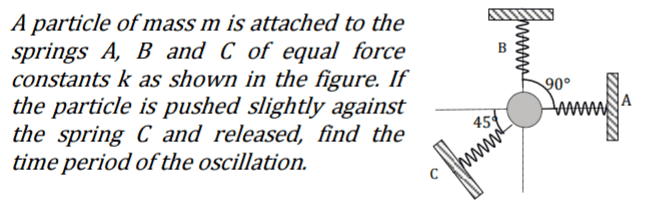# Time period of block springs SHM

## Homework Statement## The Attempt at a Solution

Length of the unstretched springs is L .Suppose block is pushed towards C by a small distance x . This causes a change in length of springs B and C . Their new length is L+∆L .Consider spring B. If the new length makes a small angle θ with the vertical .

I need to find relation between ∆L and x .

Applying cosine rule ,

x2 = (L+∆L)2 + L2 -2L(L+∆L)cosθ

For small angles , cosθ≈1.

Using this I get ∆L = x .

This is not correct . What is the mistake ?

## Answers and Replies

haruspex
Science Advisor
Homework Helper
Gold Member
2020 Award
For small angles , cosθ≈1.
Perhaps that is not exact enough here.
How about applying the cosine rule using the known angle?

•Jahnavi
Perhaps that is not exact enough here.

Could you explain what do you mean by this .

Should I use cosθ ≈ 1-θ2/2 , but then what value should I put for θ ?

haruspex
Science Advisor
Homework Helper
Gold Member
2020 Award
Should I use cosθ ≈ 1-θ2/2
Yes, but as you say the result is unhelpful, so why not try the alternative I suggested.

How about applying the cosine rule using the known angle?

That does give correct resultThank you .

This is one of the tougher problems in the book and the hint along with question is to use small angle approximation . This is why I used angle θ as it seemed to be the only small angle in the triangle formed .

If you can think of some why how to use small angle approximation , please let me know .

Last edited:
haruspex
Science Advisor
Homework Helper
Gold Member
2020 Award
how to use small angle approximation
No, it does not seem useful here.
A simpler way is to drop a perpendicular from the original position of the mass to a stretched spring and observe that this produces a roughly 45-45-90 triangle with x as the hypotenuse and ΔL on each of the other sides.

•Jahnavi
A simpler way is to drop a perpendicular from the original position of the mass to a stretched spring and observe that this produces a roughly 45-45-90 triangle with x as the hypotenuse and ΔL on each of the other sides.

This is so goodHow did you get this ? It is not obvious to me . How is length of the perpendicular ∆L (or how are the two angles 45° each ) ?

Last edited:
haruspex
Science Advisor
Homework Helper
Gold Member
2020 Award
This is so goodHow did you get this ? It is not obvious to me . How is length of the perpendicular ∆L (or how are the two angles 45° each ) ?
The perpendicular will meet the stretched spring roughly L from the spring's anchor point, leaving ΔL on the other side, from the perpendicular to the mass. Since that small triangle is nearly isosceles, the perpendicular itself is length ΔL.

•Jahnavi
The perpendicular will meet the stretched spring roughly L from the spring's anchor point,

How ?

Since that small triangle is nearly isosceles,

How ?

haruspex
Science Advisor
Homework Helper
Gold Member
2020 Award
The perpendicular will meet the stretched spring roughly L from the spring's anchor point
To be exact, L cos(θ) ≈ L.
Since that small triangle is nearly isosceles
Angles are 90, 45-θ, 45+θ.

•Jahnavi
To be exact, L cos(θ) ≈ L.

Angles are 90, 45-θ, 45+θ.

OK . Thanks